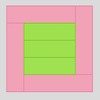#### You may also like### Cuisenaire Squares

These squares have been made from Cuisenaire rods. Can you describe the pattern? What would the next square look like?### Making Boxes

Cut differently-sized square corners from a square piece of paper to make boxes without lids. Do they all have the same volume?### A Right Charlie

Can you use this information to work out Charlie's house number?

# Augustus' Age

##### Age 7 to 11Challenge Level

Well done to Elora, Thomas, Sarah and Katie at Ysgol Gynradd Dolgellau for their solution. Again, they took a very logical approach.

We estimated an age for Augustus to start and then tried different multiplying sums until we got to the answer.

$40 \times 40=1600$

$50 \times 50=2500$

$45 \times 45=2025$

$44 \times 44=1936$

$43 \times 43=1849$

We thought that if he was $43$ in 1849 he was born in 1806 and was $65$ when he died. We thought that was reasonable.

The only way we think that someone living now would be able to say it is if someone who was born in 1980 could say in 2025 that they are $45$. A person who was $44$ in 1936 would be $109$ now and the oldest person we know of is only $101$.

Class 7P at Loretto Junior School in Musselburgh, East Lothian also worked on this problem and used a similar method. They said:

$10 \times 10 = 100$AD so he would have been born in 90 AD

Then $70 \times 70 =4900$ AD so he would be born in 4830 AD

Then $50 \times 50 = 2500$ AD so he was born in 2450 AD

Then $40 \times 40 = 1600$ AD so he was born in 1560AD

Then $43 \times 43 = 1849$ AD

So he was born in 1806. If he was born in 1806 then de Morgan would be $65$ when he died in 1871.

If you were $45$ in 2025 you would be born in 1980 and so you would be $30$ now like Euan's sister Caroline!